- 5 minutes read

# SQL Window Functions By Explanation

In our previous post, we explained how SQL window functions work by example. We started with some very simple, basic functions. Let's extend it by explaining subclauses in window functions.

SQL window functions are a great way to compute results from a set of rows rather than a single row. As you know from our first article, the "window" in window function refers to the set of rows. We showed you some examples of simple window functions like `RANK` and `LEAD`. Today, we're going to use racecars to show you how to use the `PARTITION BY` and `ORDER BY` subclauses in window functions.

That's right, we said racecars. Everyone, start your SQL engines!

### Getting Started

Window functions can only be used in the `SELECT` or `ORDER BY` clauses of a SQL query. In a window function, all the rows returned by the `SELECT` are divided into windows (or frames). The criteria for deciding which rows belong to which window is defined by putting a `PARTITION BY` subclause inside the window function invocation. The window function then computes one result based on all the rows in one window.

Let's see an example.

The following table is a subset of Formula 1 racing results for 2016 and 2017.

pilot_name circuit_name year time finish
Alonso Monza 2016 1:57:06.32 true
Hamilton Monza 2016 1:51:54.28 true
Vetel Monza 2016 1:52:04.12 true
Alonso Montecarlo 2016 0:43:14.73 false
Hamilton Montecarlo 2016 1:12:09.12 true
Vetel Montecarlo 2016 0:21:54.73 false
Raikonen Montecarlo 2016 1:14:04.12 true
Hamilton Monza 2017 1:13:16.97 true
Vetel Monza 2017 1:11:39.12 true
Raikonen Montecarlo 2017 0:43:14.73 false
Alonso Montecarlo 2017 1:32:14.42 true
Hamilton Montecarlo 2017 0:43:14.73 false
Vetel Montecarlo 2017 1:33:04.12 true

Suppose we want to create a report with the pilots' names, their time in every race (where they finished), the average time for that race, and the historical average time for that circuit. The next query will get this data for us:

```SELECT
pilot_name,
circuit_name,
year,
time,
AVG(time) OVER (PARTITION BY circuit_name) avg_this_circuit,
AVG(time) OVER (PARTITION BY circuit_name,year) avg_this_race
FROM races
WHERE finish = true
ORDER BY year desc, circuit_name, time
```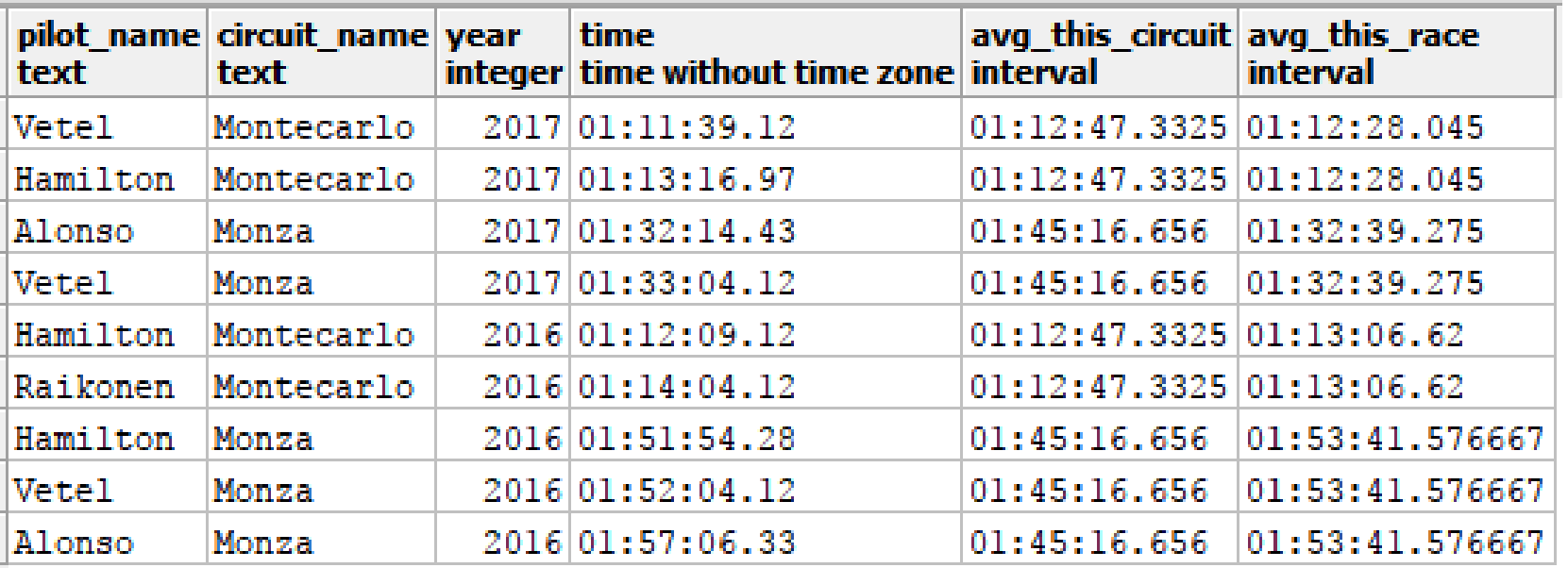To understand how the `avg_this_circuit` column was obtained, let's look at what PARTITION BY did. It generated two windows: one for Monte Carlo and the other for Monza. Then the average was calculated based on all the rows in each set. Note that there are only two different values in the `avg_this_circuit` column.

The `avg_this_race` column was generated in a similar way, except that the `PARTITION BY` divided the data into four windows: ("Montecarlo", 2017) ("Monza", 2017), ("Montecarlo", 2016) and ("Monza", 2016).

Below, you can see the complete frames for each circuit and for each circuit-year: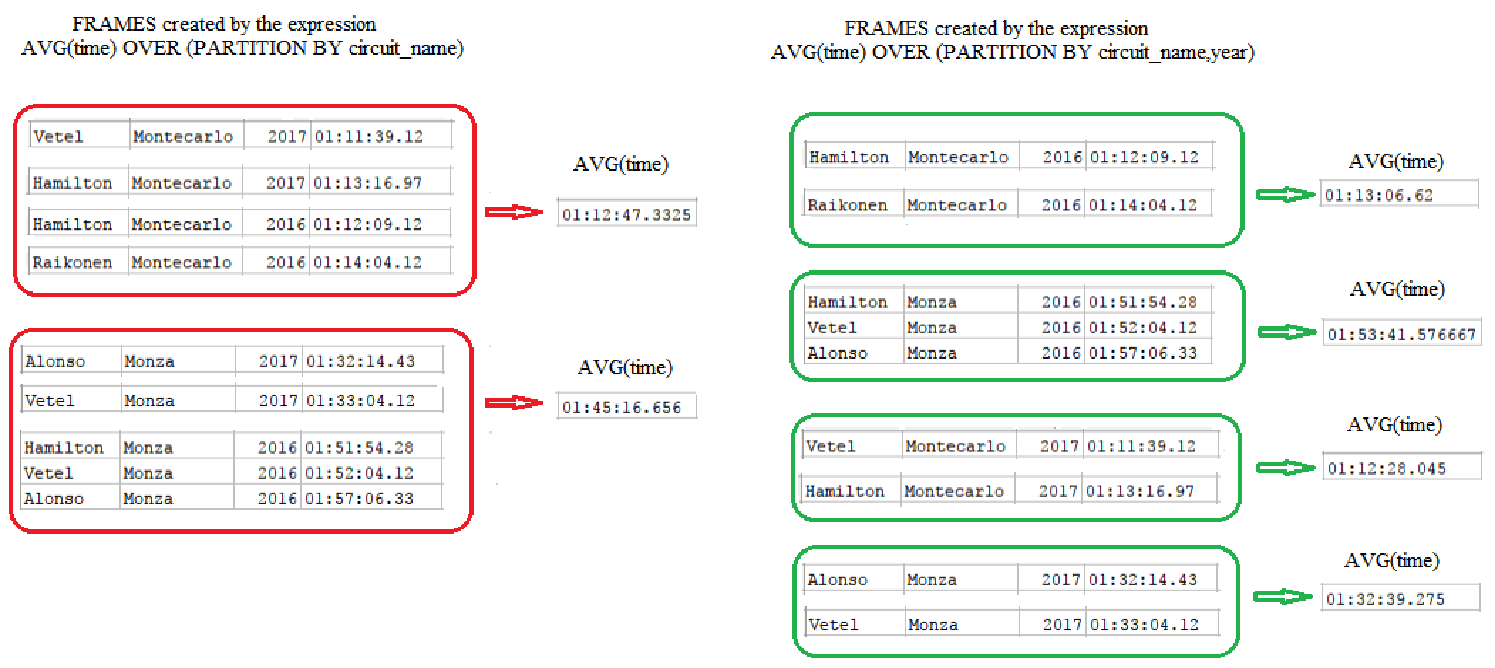### First Shall Also Be Last?

For some window functions, it is important to order the rows within the window. You can do this using the `ORDER BY` subclause.

Let's see how this works. First, we'll execute a query to obtain every pilot name, their time, their position in the race (via the `RANK` function), the time of the race winner (via the `FIRST_VALUE` function) and the delta time between this pilot and the winner. Here is the query:

```SELECT
pilot_name,
circuit_name,
year,
time pilot_time,
RANK() OVER (PARTITION BY circuit_name, year ORDER BY time) as position,
FIRST_VALUE(time) OVER (PARTITION BY circuit_name, year ORDER BY time) winner_time,
time - FIRST_VALUE(time) OVER (PARTITION BY circuit_name, year ORDER BY time) difference_time
FROM races
WHERE finish = true
ORDER BY year desc, circuit_name, time
```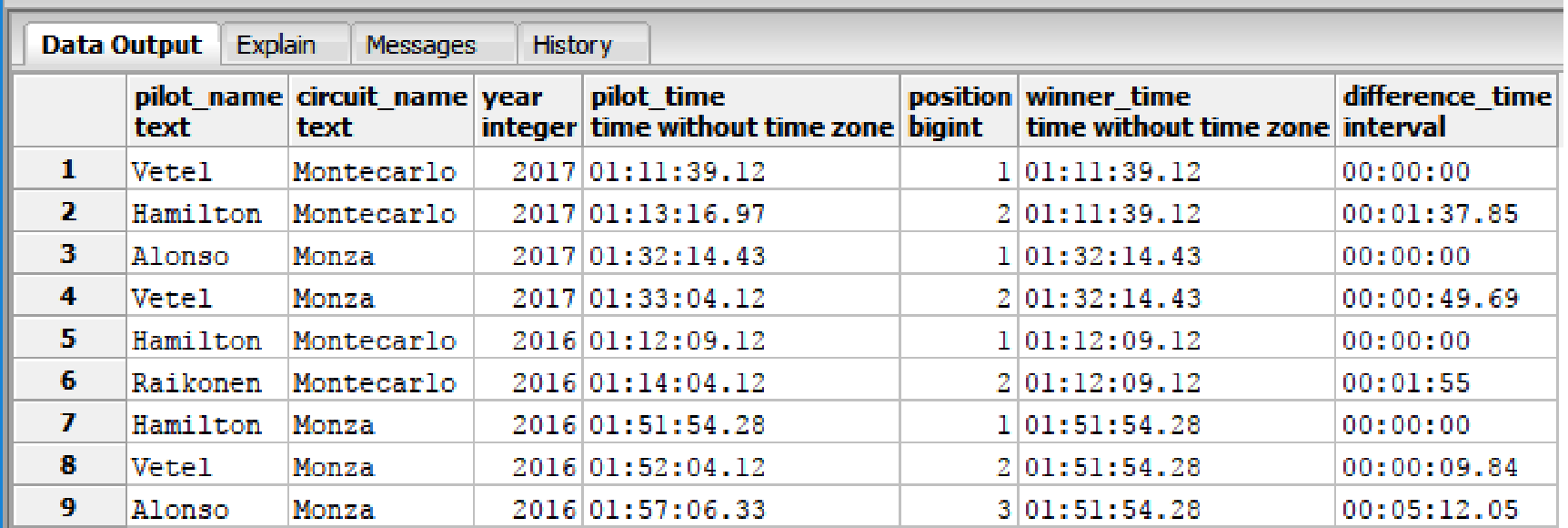Suppose we now want to add the winner's name and the name of the last pilot to finish the race. This is easy: we just add two expressions to the query. (Note: We've shown these expressions in bold text).

```SELECT
pilot_name,
circuit_name,
year,
time pilot_time,
RANK() OVER (PARTITION BY circuit_name, year ORDER BY time) as position,
FIRST_VALUE(pilot_name) OVER (PARTITION BY circuit_name, year ORDER BY time) winner_pilot,
LAST_VALUE(pilot_name) OVER (PARTITION BY circuit_name, year ORDER BY time) last_pilot
FROM races
WHERE finish = true
ORDER BY year desc, circuit_name, time
```

And here are the results: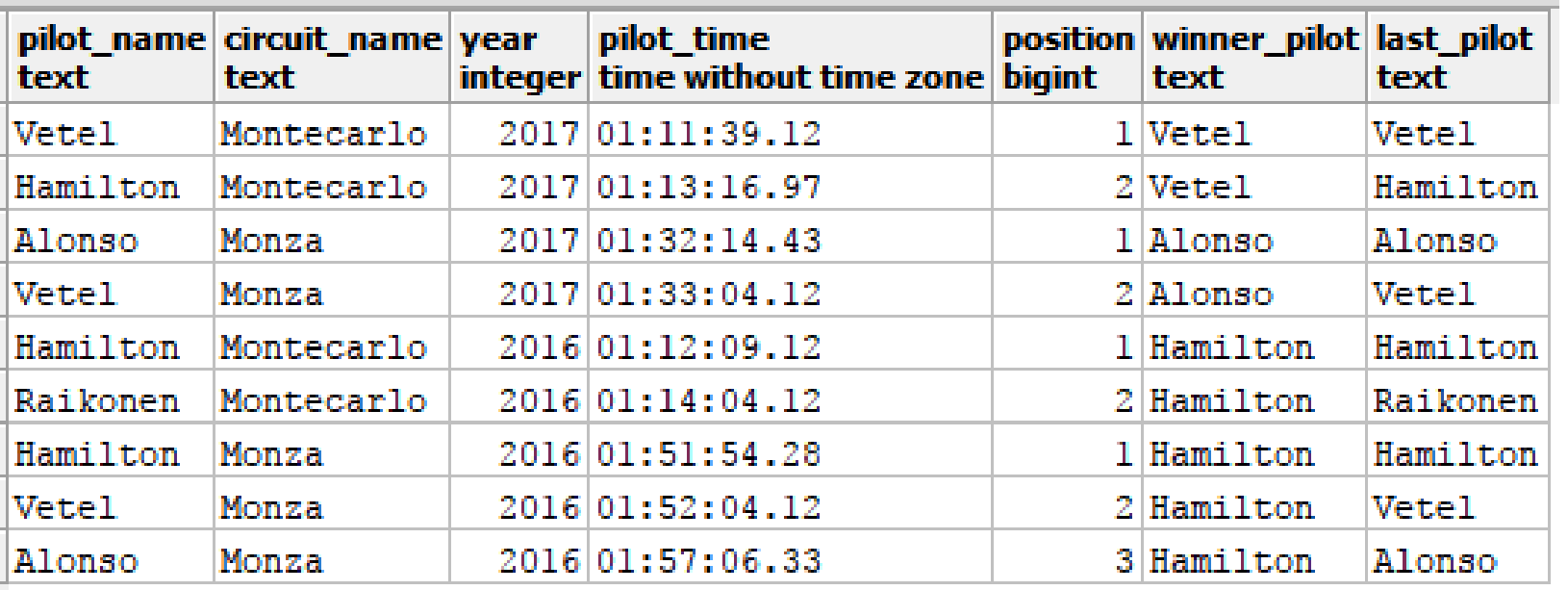If we analyze the query results, we will find that the winner column is correct, but the `last_pilot` column is not. In fact, they show the same name: Vatel. How did this happen?

Remember, the window function is calculated over a set of rows. It is dynamic, changing with every row returned by the `SELECT`. At every moment when the query is working, there is a current row in the result set and the window is formed by all the rows in the partition except any rows yet to be returned. When we calculate the `last_pilot` column, we are taking the last value in the window, which corresponds to the current row being added to the frame.

The following image visualizes this scenario: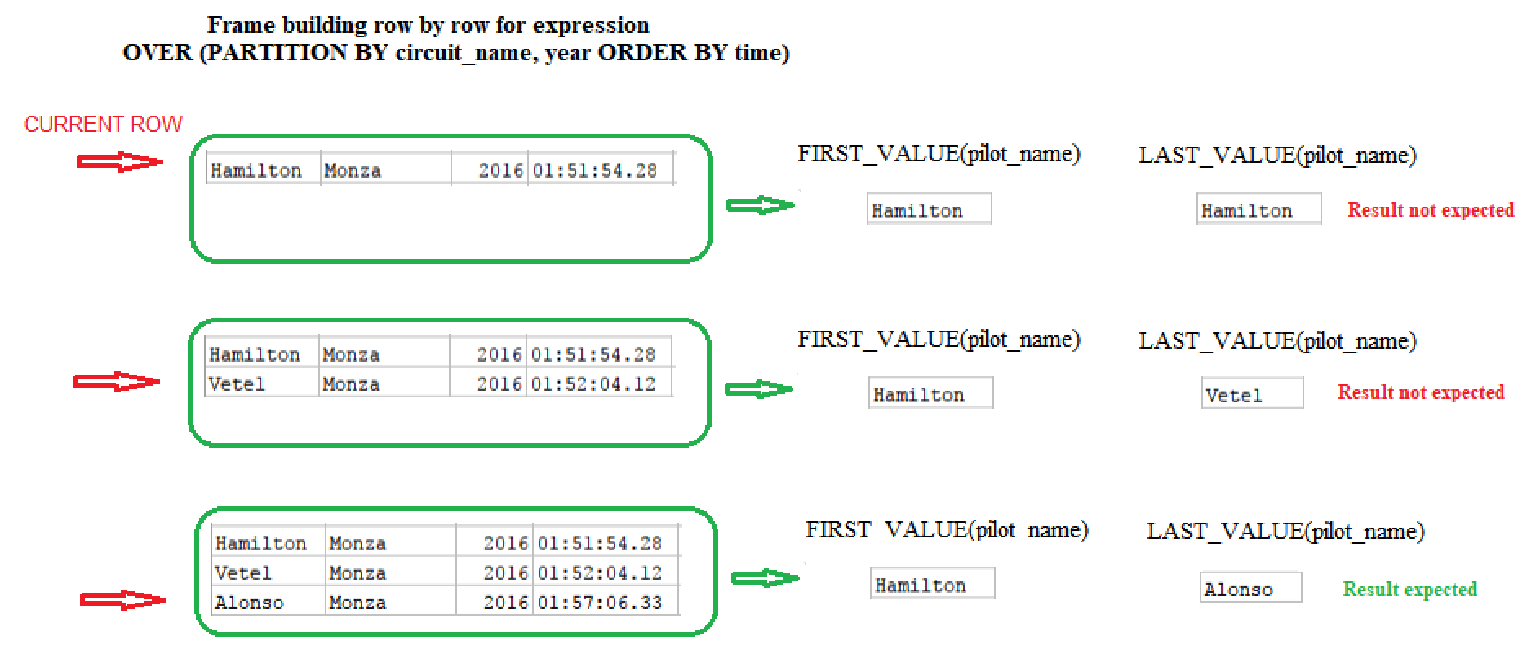### Fixing the Problem

The previous issue with `last_pilot` column is due to the `FIRST_VALUE`, `LAST_VALUE`, and `NTH_VALUE` functions. These consider only the rows within the window frame, which only contains rows up to the current row. Thus, `LAST_VALUE` does not return the correct value. In order to fix this, we need to add a frame specification (`RANGE` or `ROWS`) to the `OVER` clause.

We can select a different range in the partition by using another subclause. Some of the most important options are:

• `ROWS BETWEEN UNBOUNDED PRECEDING AND CURRENT ROW (default)`
• `ROWS BETWEEN CURRENT ROW AND UNBOUNDED FOLLOWING`
• `RANGE BETWEEN UNBOUNDED PRECEDING AND UNBOUNDED FOLLOWING`

We would add this subclause to the ORDER BY clause. Let's see how the first option works:

```SELECT
pilot_name,
circuit_name,
year,
time pilot_time,
rank() OVER (PARTITION BY circuit_name, year ORDER BY time) as position,
FIRST_VALUE(pilot_name) OVER (PARTITION BY circuit_name, year ORDER BY time) winner_pilot,
LAST_VALUE(pilot_name)
OVER (PARTITION BY circuit_name, year
ORDER BY time ROWS BETWEEN CURRENT ROW AND UNBOUNDED FOLLOWING ) last_pilot
FROM races
WHERE finish = true
ORDER BY year desc, circuit_name, time
```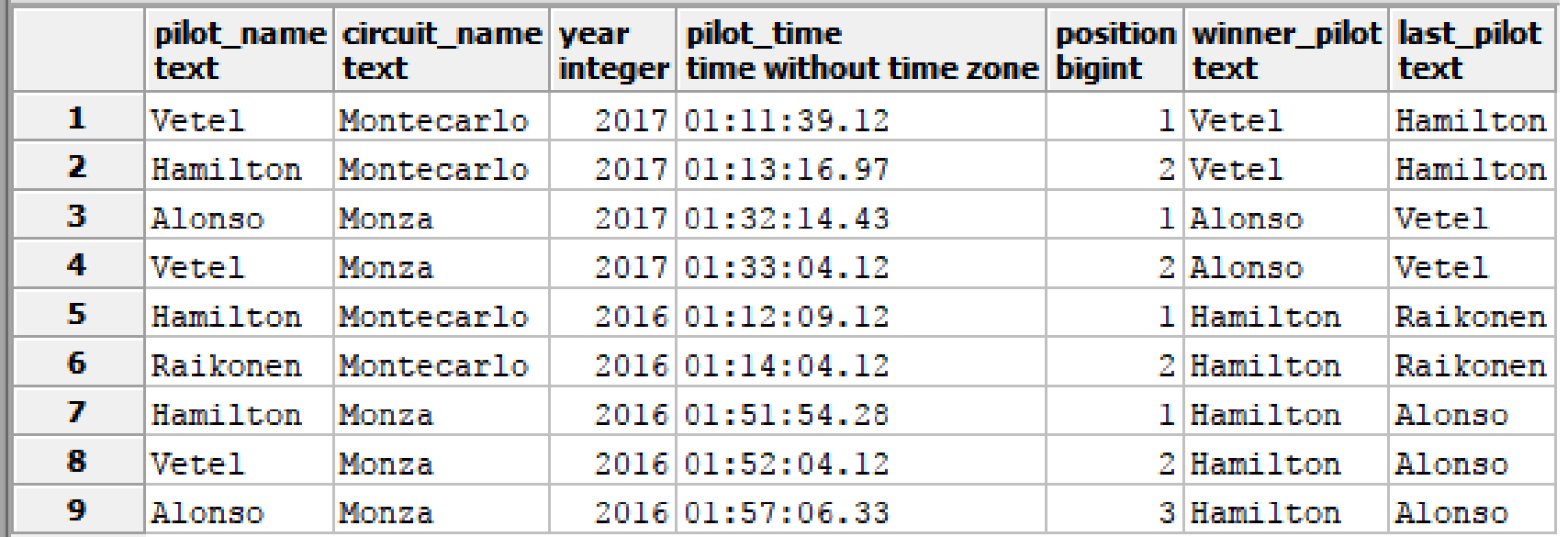It worked!

### Learning More About SQL Window Functions

This was just a short example of how we can use subclauses to power up SQL window functions. These might be one of the least-known parts of SQL, but they are powerful, flexible, and worth spending time to master. You can learn more about SQL window functions on our blog, or sign up for the Window Functions course to really dig into the subject!